#### STAT 804: Lecture 22 Notes

Properties of the Periodogram

The discrete Fourier transform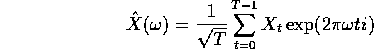is periodic with period 1 because all the exponentials have period 1. Moreover,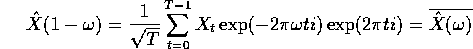so that the periodogram satisfies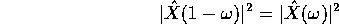Thus the periodogram is symmetric around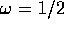which is called the Nyquist or folding frequency. (The value is always 1/2 in cycles per point but usually it would be converted to cycles per time unit like year or day or whatever.)

Similarly the power spectral density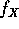given by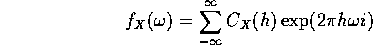is periodic with period 1 and satisfies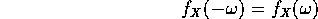which is equivalent to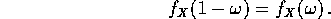Spectra of Some Basic Processes

Here I compute the spectra of a few basic processes directly and then indirectly by a more powerful technique.

Direct from the definition

White Noise:
Since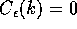for all non-zero k we have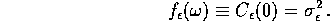MA(1):
For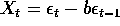we have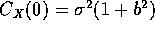and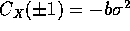so that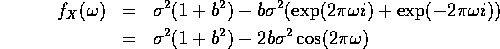AR(1):
We have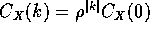and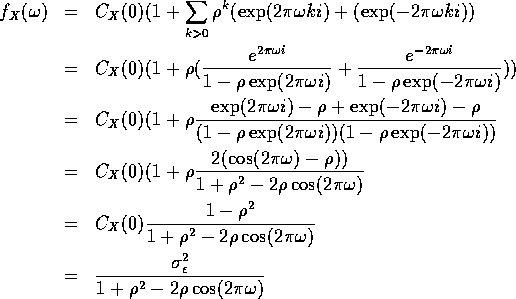Using filters

Any mean 0 ARMA(p,q) process can be rewritten in MA form as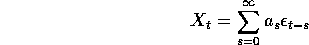and then the covariance of X is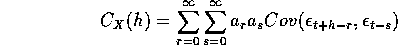Although the covariance simplifies for white noise, let us simply write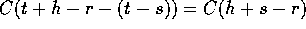for the covariance in this double sum so that the calculation will apply to any stationary. Then plug this double sum into the definition ofto get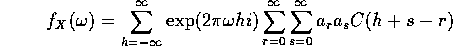Now write the h in the complex exponential in the form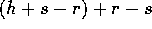and bring the sum over h to the inside to get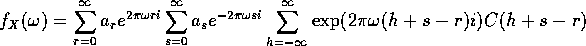Finally make the substitution k=h+s-r in the inside sum and define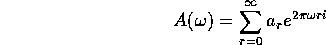to see that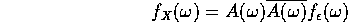or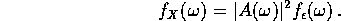The function A (or) is called the frequency response function and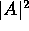the power transfer function. The jargon gain is sometimes used for |A|.

The Spectrum of an ARMA(p,q)

An ARMA(p,q) process X satisfies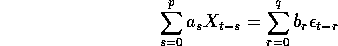so that if Y is the process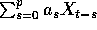then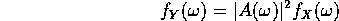where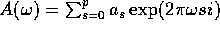. At the same time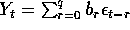so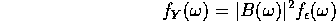where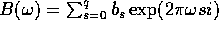. Hence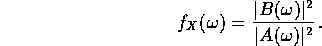For example, in the ARMA(1,1) case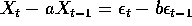we find (referring to our MA(1) calculation above that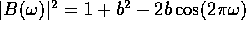and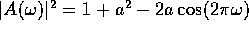so that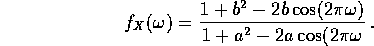Richard Lockhart
Wed Nov 19 13:55:49 PST 1997